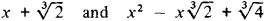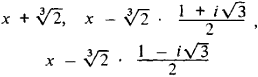# Irreducible Polynomial

Also found in: Wikipedia.

## irreducible polynomial

[‚ir·ə′dü·sə·bəl ‚päl·ə′nō·mē·əl]
(mathematics)
A polynomial is irreducible over a field K if it cannot be written as the product of two polynomials of lesser degree whose coefficients come from K. Also known as irreducible function.
McGraw-Hill Dictionary of Scientific & Technical Terms, 6E, Copyright © 2003 by The McGraw-Hill Companies, Inc.
The following article is from The Great Soviet Encyclopedia (1979). It might be outdated or ideologically biased.

## Irreducible Polynomial

a polynomial that cannot be factored into factors of lower degree. The possibility of factoring a polynomial into factors and the irreducibility property depend on the numbers that can be coefficients of the polynomial. Thus, the polynomial x3 + 2 is irreducible if only rational numbers are admitted as coefficients; but it is factored into the product of two irreducible polynomialsif any real numbers can be taken as the coefficients and into the product of three factorsif complex numbers are the coefficients. In the general case, the concept of irreducibility is defined for polynomials with coefficients belonging to an arbitrary field. A polynomial with rational coefficients that cannot be factored into factors of lower degree with rational coefficients is often called an irreducible polynomial.

### REFERENCE

Kurosh, A. G. Kurs vysshei algebry, 9th ed. Moscow, 1968.